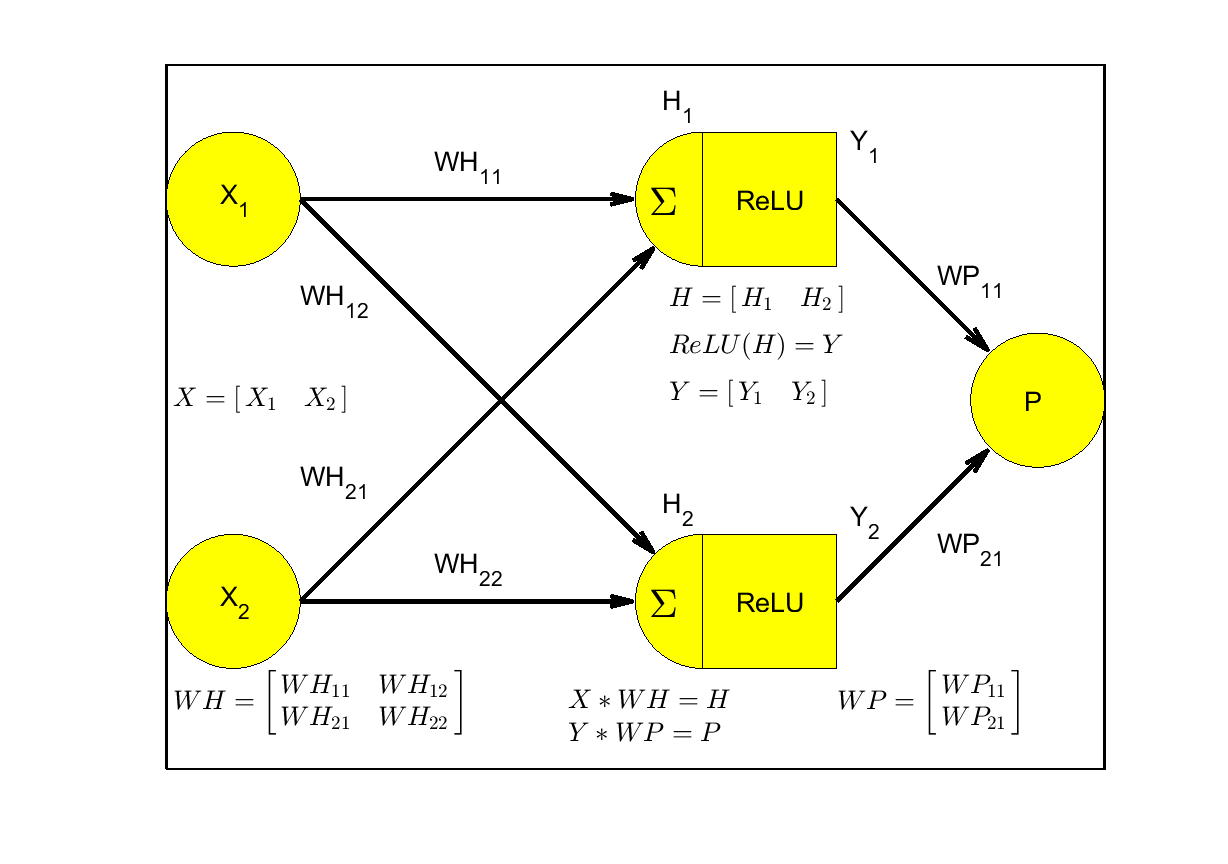# Problem 58892. Neural Net: Calculate Perceptron

This challenge is to calculate the Neural Net Perceptron value,P, given X, WH, and WP using ReLU on the hidden layer. This example is for two inputs, two hidden nodes, and a single output. No offset terms are applied.The function template includes the code to create this figure using Matlab Latex

### Solution Stats

100.0% Correct | 0.0% Incorrect
Last Solution submitted on Sep 25, 2023

### Community Treasure Hunt

Find the treasures in MATLAB Central and discover how the community can help you!

Start Hunting!